# Fluctuation

(redirected from fluctuancy)
Also found in: Dictionary, Thesaurus, Medical, Financial.

## fluctuation

[‚flək·chə′wā·shən]
(oceanography)
Wavelike motion of water.
The variations of water-level height from mean sea level that are not due to tide-producing forces.
(science and technology)
Variation, especially back and forth between successive values in a series of observations.
Variation of data points about a smooth curve passing among them.

## Fluctuation

a random deviation of an observed physical quantity from its average value. Fluctuations occur in any quantities that depend on random factors and that can be described by statistical methods (see).

The quantitative description of fluctuations is based on the methods of mathematical statistics and probability theory. The simplest measure of the fluctuation of a quantity x is the quantity’s variance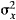—the mean square deviation of x from its average value x̄: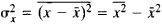where a bar over a quantity signifies statistical averaging. An equivalent measure of fluctuations is the standard deviation σx, which is the square root of the variance; the relative value σx = σx/x̄ may also be used.

In statistical mechanics the observed values of physical quantities are very close to the quantities’ average statistical values. In other words, the fluctuations caused by the random thermal motion of particles—such as fluctuations of average energy, density, or pressure—are very small. They are of fundamental importance, however, since they limit the range of applicability of thermodynamic concepts to large systems (that is, systems containing many particles), for which the fluctuations are much smaller than the fluctuating quantities. The existence of fluctuations makes possible a more precise understanding of the second law of thermodynamics. It remains true, however, that a perpetual motion machine of the second kind is impossible: although a system may undergo fluctuations from an equilibrium state to nonequilibrium states that have lower entropy, a perpetual motion machine of the second kind cannot be constructed on the basis of such fluctuations. The principle of entropy increase in an isolated system remains valid for average quantities.

The foundations of the theory of fluctuations were laid by J. Gibbs, A. Einstein, and M. Smoluchowski.

By using Gibbs distributions it is possible to calculate the fluctuations in a state of statistical equilibrium for systems that are under different physical conditions. Here, the fluctuations are expressed in terms of equilibrium thermodynamic parameters and the derivatives of thermodynamic potentials.

Consider, for example, a system with constant volume V and a constant number of particles N. If the system is in contact with a heat reservoir that is at temperature T, the Gibbs canonical distribution gives for energy E the mean square fluctuation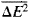= (kT)2CV, where k is the Boltzmann constant and CV is the specific heat at constant volume. Such an expression for fluctuations is valid in the case of quantum statistics as well; only the explicit expressions for CV differ.

For systems with constant volume in contact with a heat reservoir and a reservoir of particles, the Gibbs grand canonical distribution yields for the number of particles the mean square fluctuation, where μ is the chemical potential.

In the two examples cited, quantities proportional to the volume (that is, extensive quantities) fluctuate. Their relative mean square fluctuations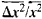are proportional to the quantity I/N and consequently are very small. Such fluctuations are said to be normal. At phase transition points fluctuations increase markedly, and their relative decrease with N may be slower.

To give a more detailed description of fluctuations, it is necessary to know their probability distribution function. The probability w(x1,. . ., xn) of the fluctuation of some quantities x1 . . ., xn from a state of incomplete thermodynamic equilibrium with entropy S(1, . . ., n) to a state with entropy S(x1, . . .,xn) is determined by Boltzmann’s formula

w(x1,...,xn)/w(1,...,n) = exp {S(x1,...,xn) – S(1,...,n)}

since the entropy is equal to the logarithm of the statistical weight, that is, of the thermodynamic probability of the state. By the entropy of a state of incomplete equilibrium is meant the entropy of an auxiliary equilibrium state that is characterized by the same average values of xi as the given nonequilibrium state. For small Δxi = xii the formula turns into the Gaussian distribution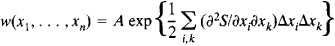where A is a constant determined from the probability normalization condition.

It is possible to find not only the fluctuations of quantities xi but also the correlations between fluctuations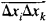, which define the interrelation of the fluctuations; it may be noted that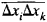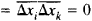only in the case of statistically independent quantities. Thus, we have the correlation between temperature and pressure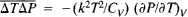(the temperature is related to the average energy) and the correlation between volume and pressure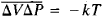. In general, for the physical quantities A(x, t) and B(x, t), which depend on the coordinates x and time t, correlations obtain between their fluctuations at different points of space and at different times: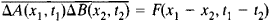The functions F are sometimes called space-time correlation functions and in a state of statistical equilibrium depend solely on the differences in coordinates and time. For the particle density n the functionscan be experimentally measured from the scattering of slow neutrons or X rays. The second derivative of the neutron scattering cross section determines the Fourier transform of the space-time correlation function of particle densities in a medium.

Fluctuations are associated with nonequilibrium processes. Such nonequilibrium characteristics of a system as the kinetic coefficients (seeKINETICS, PHYSICAL) are proportional to the integrals over time of the time correlation functions of fluxes of physical quantities (the Kubo-Green formulas). For example, the electrical conductivity is proportional to the integral of the correlation functions of current densities, and the coefficients of thermal conductivity, viscosity, and diffusion are proportional to the integrals of the correlation functions of heat, momentum, and diffusion flux densities, respectively.

In the general case a relation holds between the fluctuations of physical quantities and the dissipative properties of a system under the action of an external disturbance. According to the fluctuation-dissipation theorem, the reaction of a system to some disturbance—that is, the corresponding change in some physical quantity—is determined by the generalized susceptibility, the imaginary part of which is proportional to the Fourier component of the time correlation function of the disturbances associated with the given influence.

Fluctuations in systems of charged particles are manifested as random changes in potentials, currents, or charges. They are due both to the discreteness of the electric charge and to the thermal motion of the charge carriers. These fluctuations are the cause of electrical noise and set a limit on the sensitivity of instruments for recording weak electric signals.

Fluctuations can be observed through the scattering of light. The random changes produced in the density of a medium by fluctuations cause random changes in the refractive index; consequently, light can be scattered not only in a turbid medium but also in a medium of uniform composition or even in a chemically pure substance. This phenomenon is especially noticeable in binary solutions at a temperature close to the critical separation temperature; the term “critical scattering” is used in this case. Fluctuations are also very great at the critical point of liquidvapor equilibrium (seeCRITICAL PHENOMENA). Pressure fluctuations in a gas or liquid show up in the Brownian movement of small particles suspended in the fluid; the movement occurs because the impacts of the medium’s molecules on a particle do not counterbalance one another exactly.

### REFERENCES

Einstein, A., and M. Smoluchowski. Braunovskoe dvizhenie: Sb. Moscow-Leningrad, 1936. (Translated from German.)
Leontovich, M. A. Statisticheskaia fizika. Moscow-Leningrad, 1944.
Munster, A. “Teoriia fluktuatsii.” In the collection Termodinamika neobratimykh protsessov. Moscow, 1962. (Translated from English.)
Zubarev, D. N. Neravnovesnaia statisticheskaia termodinamika. Moscow, 1971.
Levin, M. L., and S. M. Rytov. Teoriia ravnovesnykh teplovykh fluktuatsii v elektrodinamike. Moscow, 1967.

D. N. ZUBAREV

Site: Follow: Share:
Open / Close